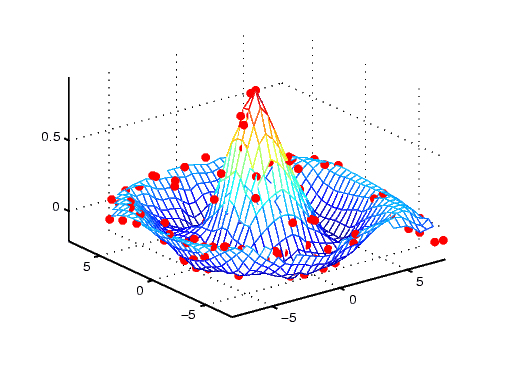3-D VisualizationSurface Plots of Nonuniformly Sampled Data

You can use `meshgrid` to create a grid of uniformly sampled data points at which to evaluate and graph the `sinc` function. MATLAB then constructs the surface plot by connecting neighboring matrix elements to form a mesh of quadrilaterals.

To produce a surface plot from nonuniformly sampled data, first use `griddata` to interpolate the values at uniformly spaced points, and then use `mesh` and `surf` in the usual way.

Example -- Displaying Nonuniform Data on a Surface

This example evaluates the `sinc `function at random points within a specific range and then generates uniformly sampled data for display as a surface plot. The process involves these tasks:

• Use `linspace` to generate evenly spaced values over the range of your unevenly sampled data.
• Use `meshgrid` to generate the plotting grid with the output of `linspace`.
• Use `griddata` to interpolate the irregularly sampled data to the regularly spaced grid returned by `meshgrid`.
• Use a plotting function to display the data.
1. First, generate unevenly sampled data within the range [-8, 8] and use it to evaluate the function.
• ```x = rand(100,1)*16 - 8;
y = rand(100,1)*16 - 8;
r = sqrt(x.^2 + y.^2) + eps;
z = sin(r)./r;
```
2. The `linspace` function provides a convenient way to create uniformly spaced data with the desired number of elements. The following statements produce vectors over the range of the random data with the same resolution as that generated by the -8:.5:8 statement in the previous `sinc` example.
• ```xlin = linspace(min(x),max(x),33);
ylin = linspace(min(y),max(y),33);
```
3. Now use these points to generate a uniformly spaced grid.
• ```[X,Y] = meshgrid(xlin,ylin);
```
4. The key to this process is to use `griddata` to interpolate the values of the function at the uniformly spaced points, based on the values of the function at the original data points (which are random in this example). This statement uses a triangle-based cubic interpolation to generate the new data.
• ```Z = griddata(x,y,z,X,Y,'cubic');
```
5. Plotting the interpolated and the nonuniform data produces
• ```mesh(X,Y,Z) %interpolated
axis tight; hold on
plot3(x,y,z,'.','MarkerSize',15) %nonuniform```Visualizing Functions of Two Variables Parametric Surfaces© 1994-2005 The MathWorks, Inc.